https://doi.org/10.5194/bg-16-3651-2019
https://doi.org/10.5194/bg-16-3651-2019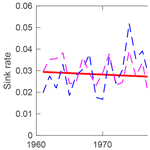# Trend analysis of the airborne fraction and sink rate of anthropogenically released CO2

Mikkel Bennedsen, Eric Hillebrand, and Siem Jan Koopman
Abstract

Is the fraction of anthropogenically released CO2 that remains in the atmosphere (the airborne fraction) increasing? Is the rate at which the ocean and land sinks take up CO2 from the atmosphere decreasing? We analyse these questions by means of a statistical dynamic multivariate model from which we estimate the unobserved trend processes together with the parameters that govern them. We show how the concept of a global carbon budget can be used to obtain two separate data series measuring the same physical object of interest, such as the airborne fraction. Incorporating these additional data into the dynamic multivariate model increases the number of available observations, thus improving the reliability of trend and parameter estimates. We find no statistical evidence of an increasing airborne fraction, but we do find statistical evidence of a decreasing sink rate. We infer that the efficiency of the sinks in absorbing CO2 from the atmosphere is decreasing at approximately 0.54 % yr−1.

Share
Dates
1 Introduction

A part of the anthropogenically released CO2 emitted to the atmosphere flows to the oceans (the ocean sink) and the terrestrial biosphere (the land sink). Approximately 45 % of released CO2 stays in the atmosphere (the airborne fraction), while the two sinks take up approximately 24 % and 31 % of the CO2, respectively. (These percentages are calculated over the period 1959 to 2016 using the data described below; see, for example, Raupach et al.2014, for similar estimates.) A key question is whether the airborne fraction is increasing or if it remains constant at around 45 %. An increasing airborne fraction implies that the share of anthropogenically released CO2 that ultimately remains in the atmosphere increases, and projections of future atmospheric CO2 levels need to take this into account . Closely related is the question of whether the sinks will continue taking up CO2 at the same rate (the sink rate) or if this rate is decreasing. A decreasing sink rate implies that the efficiency with which ocean and land sinks are absorbing CO2 from the atmosphere is decreasing. Thus, analysing the behaviour of the sink rate can help predict the future uptake of CO2 through the ocean and the land sink. The answers to the questions posed above are important for our understanding of the global carbon cycle and are relevant for policymakers and the public in general.

A series of papers argue that the airborne fraction of anthropogenically released CO2 (mainly through fossil fuel emissions, cement production, and land-use change) is increasing . Similarly, in , it is argued that, although the statistical evidence of an increasing airborne fraction is relatively weak, the evidence of a decreasing CO2 sink rate is clearer. However, the methods in these studies have been criticized in, for example, , , and . Indeed, by considering a longer data set and incorporating uncertainties into the data, found that the conclusion of an increasing airborne fraction was not warranted. Similarly, argues that errors in the data can lead to erroneous conclusions regarding possible trends in the airborne fraction and in the sink rate.

In this paper, we address these statistical issues within the framework of a state-space system. It allows us to conduct statistical inference by taking explicit account of stochastic and deterministic trends in the data, transient shocks to the data (coming from, e.g. volcanic eruptions or strong El Niño events), and (potential) measurement errors. It also allows for the simultaneous incorporation of multiple data sets for the same object, which can improve the estimation of trends and increase reliability of parameter estimates. We find strong evidence for purely deterministic trends when we incorporate multiple measurements for the airborne fraction and the sink rate. These deterministic trends have a statistically significantly negative slope in the case of the sink rate and an insignificant slope in the case of the airborne fraction. These findings corroborate earlier findings in the literature, especially those of , but address the statistical concerns raised by and , among others. Finally, by analysing the ocean and land sink rates separately, we find no evidence of a decreasing ocean sink rate, but we do find evidence that the land sink rate is decreasing.

The paper is organized as follows. In Sect. 2 we state the fundamental equations of the global carbon budget, the definitions of the airborne fraction of anthropogenically released CO2, and the CO2 sink rate, which will motivate the specification of the state-space system. Section 3 introduces the state-space system used in the paper. In Sect. 4 we conduct a trend analysis of the airborne fraction within the proposed statistical framework. In Sect. 5 we carry out the corresponding analysis of the CO2 sink rate, and in Sect. 6 we carry out the analysis of the land and ocean sink rates separately. Section 7 discusses the results, and Sect. 8 concludes the paper. The Supplement is available online.

2 The global carbon budget

The so-called global carbon budget is defined as

$\begin{array}{}\text{(1)}& {E}_{t}^{\mathrm{ANT}}={G}_{t}+{S}_{t}^{O}+{S}_{t}^{L},\end{array}$

where ${E}_{t}^{\mathrm{ANT}}$ is anthropogenically released CO2 into the atmosphere, Gt is growth of atmospheric CO2 concentration, ${S}_{t}^{O}$ is the flux of CO2 from the atmosphere to the oceans (the ocean sink), and ${S}_{t}^{L}$ is the flux of CO2 from the atmosphere to the terrestrial biosphere (the land sink). In other words, Eq. (1) states that emissions of CO2 should equal the fluxes of CO2 to the atmosphere, the ocean sink, and the land sink. We use the data set provided by the Global Carbon Project .1 All data are given in gigatonnes of carbon (GtC) and are recorded at a yearly frequency, beginning in 1959 and ending in 2016, resulting in 58 observations for each quantity in Eq. (1).

While the carbon budget is in principle always balanced for the physical quantities, in the sense that Eq. (1) always holds, this might not be the case when inserting actual data for emissions and sinks due to measurement errors in the data. For this reason, introduce a residual term into the budget Eq. (1) to capture measurement error. It is denoted ${B}_{t}^{\mathrm{IM}}$ for budget imbalance. Therefore, when considering actual data, the carbon budget is defined as

$\begin{array}{}\text{(2)}& {E}_{t}^{\mathrm{ANT}}={G}_{t}+{S}_{t}^{O}+{S}_{t}^{L}+{B}_{t}^{\mathrm{IM}}.\end{array}$

The sample mean of the budget imbalance over the observation period is not significantly different from zero and shows no sign of a trend . These facts are important in the developments below, since they motivate treating ${B}_{t}^{\mathrm{IM}}$ as part of an error term.

The growth rate in atmospheric CO2 data, Gt, is from , the ocean sink data, ${S}_{t}^{O}$, are obtained from an ensemble of global biochemistry models, and the land sink data, ${S}_{t}^{L}$, are estimated as the multi-model mean of several dynamic global vegetation models. See for further information on the data. The anthropogenic emissions of CO2 can be decomposed into two parts:

${E}_{t}^{\mathrm{ANT}}={E}_{t}^{\mathrm{FF}}+{E}_{t}^{\mathrm{LUC}},$

where ${E}_{t}^{\mathrm{FF}}$ are emissions from fossil fuel burning, cement production, and gas flaring, while ${E}_{t}^{\mathrm{LUC}}$ are emissions from land-use change. Fossil fuel emissions, ${E}_{t}^{\mathrm{FF}}$, are from , while land-use change emissions, ${E}_{t}^{\mathrm{LUC}}$, are averages of the results of the two bookkeeping models of and , updated as in . The time series of concentrations (above pre-industrial levels) of CO2 in the atmosphere is constructed as

${C}_{t}=\mathrm{2.127}\cdot \left(\left[{\mathrm{CO}}_{\mathrm{2}}{\right]}_{\mathrm{1959}}-\left[{\mathrm{CO}}_{\mathrm{2}}{\right]}_{\mathrm{1750}}\right)+\sum _{\mathit{\tau }=\mathrm{1}}^{t}{G}_{\mathit{\tau }},$

where [CO2]1750=279 ppmv (parts per million by volume) and [CO2]1959=315.39 ppmv are the concentrations of CO2 in the atmosphere in 1750 and 1959, respectively; see . The number 2.127 is the conversion factor from parts per million by volume to gigatonnes of carbon. In other words, the atmospheric concentration Ct above pre-industrial levels is given by the initial value in 1959 plus the cumulative sum of the growth in atmospheric CO2 concentrations Gt, which result from the budget Eq. (1).

We follow and and define the airborne fraction as

${\mathrm{AF}}_{t}=\frac{{G}_{t}}{{E}_{t}^{\mathrm{ANT}}},$

and the CO2 sink rate as

$\begin{array}{}\text{(3)}& {k}_{\mathrm{S},t}=\frac{{S}_{t}^{O}+{S}_{t}^{L}}{{C}_{t}},\end{array}$

which is the flux of CO2 from the atmosphere to the sinks (ocean plus land), normalized by the amount of CO2 (above pre-industrial levels) currently in the atmosphere. We can also consider the individual components of the sink rate for ocean and land, which are given by

$\begin{array}{}\text{(4)}& {k}_{O,t}=\frac{{S}_{t}^{O}}{{C}_{t}},\phantom{\rule{2em}{0ex}}{k}_{L,t}=\frac{{S}_{t}^{L}}{{C}_{t}},\end{array}$

respectively, with ${k}_{\mathrm{S},t}={k}_{O,t}+{k}_{L,t}$.

The airborne fraction and the sink rate are fundamentally different quantities. The airborne fraction AF${}_{t}={G}_{t}/{E}_{t}^{\mathrm{ANT}}$ is the ratio of the growth of atmospheric CO2 in period t to the amount of CO2 emitted in period t. It is thus a measure of the fraction of emitted CO2 that stays in the atmosphere. In contrast, the sink rate ${k}_{\mathrm{S},t}=\left({S}_{t}^{O}+{S}_{t}^{L}\right)/{C}_{t}$ is the ratio of the CO2 flux in the sinks in period t to the total amount of CO2 in the atmosphere (above pre-industrial levels). By writing ${S}_{t}^{O}+{S}_{t}^{L}={k}_{\mathrm{S},t}{C}_{t}$, we can interpret the sink rate kS,t as the “efficiency” with which CO2 flows from the atmosphere to the sinks, i.e. as the amount of CO2 going into the sinks for an extra unit of CO2 added to the atmosphere . We discuss the relationship between the airborne fraction and the sink rate further in Sect. 7.

3 Trend model specification

In this section, we consider several models for the data-generating process behind observations of the objects of interest defined in Sect. 2. Common to all models is that they can be cast in a state-space system of the form

$\begin{array}{}\text{(5)}& \begin{array}{rl}& {\mathbit{y}}_{t}=\mathbf{A}\phantom{\rule{0.125em}{0ex}}{\mathbit{x}}_{t}+{\mathbit{\xi }}_{t},\\ & {\mathbit{x}}_{t+\mathrm{1}}=\mathbf{B}\phantom{\rule{0.125em}{0ex}}{\mathbit{x}}_{t}+{\mathbit{\kappa }}_{t},\end{array}\end{array}$

where yt is a vector of observations at time $t=\mathrm{1},\mathrm{\dots },n$ with time series length n, and the system matrices A and B have appropriate dimensions. The vector xt is usually referred to as the state vector, which can include deterministic and stochastic trends, and the error terms ξt and κt are both independent and identically distributed (iid) random vectors of appropriate dimensions and with a mean of zero. For example, when we need to model the airborne fraction alone, we have yt=AFt, and the state-space system represents a univariate dynamic model for the airborne fraction. When modelling the ocean and land sink rates jointly, we have ${\mathbit{y}}_{t}=\left({k}_{O,t},\phantom{\rule{0.125em}{0ex}}{k}_{L,t}{\right)}^{\prime }$, and the state-space system is a bivariate dynamic model. For given matrices A and B, and under the assumption of mutually and serially uncorrelated Gaussian errors ξt and κt (with their respective variance matrices Σξ and Σκ), the state-space system is a linear Gaussian model. In such regular cases, an analytic formulation for the likelihood function is available and relies on the prediction error decomposition. Hence the parameters (variances and possibly covariances in Σξ and Σκ) can be estimated by the maximum likelihood method. It requires the numerical optimization of the log-likelihood function that is evaluated via the Kalman filter. The resulting algorithm is initialized with specific starting values; we use a diffuse initialization as outlined in Chapter 5 of . The smooth estimate of the state process xt can also be obtained by means of the Kalman filter together with a smoothing algorithm. The extracted state is effectively the conditional mean $\mathbb{E}\left({\mathbit{x}}_{t}|{\mathbit{y}}_{\mathrm{1}},\mathrm{\dots },{\mathbit{y}}_{n};\mathbf{A},\mathbf{B},{\mathbf{\Sigma }}_{\mathit{\xi }},{\mathbf{\Sigma }}_{\mathit{\kappa }}\right)$, for $t=\mathrm{1},\mathrm{\dots },n$. Details of the state-space approach are given by , where both signal extraction and maximum likelihood estimation are discussed.

Our baseline model is the local linear trend (LLT) model. For a univariate time series yt, we treat the underlying trend Tt as a stochastic process given by

$\begin{array}{}\text{(6)}& {T}_{t+\mathrm{1}}={T}_{t}+\mathit{\beta }+{\mathit{\eta }}_{t},\end{array}$

where β∈ℝ is a fixed and unknown coefficient and ηt is an iid Gaussian random variable with a mean of zero and variance ${\mathit{\sigma }}_{\mathit{\eta }}^{\mathrm{2}}$. The solution to the difference equation in Eq. (6) is given as

${T}_{t+\mathrm{1}}={T}_{\mathrm{1}}+t\mathit{\beta }+\sum _{i=\mathrm{0}}^{t-\mathrm{1}}{\mathit{\eta }}_{t-i},\phantom{\rule{2em}{0ex}}t=\mathrm{1},\mathrm{2},\mathrm{\dots },n-\mathrm{1},$

where T1 can be treated as a fixed unknown coefficient (intercept or constant) or as a random variable. The solution shows that the trend component is made up of the starting value T1, a deterministic linear term with slope β, and a random-walk component ${\sum }_{i=\mathrm{0}}^{t-\mathrm{1}}{\mathit{\eta }}_{t-i}$. Thus, Tt can be interpreted as a long-term trend in the time series and β as the slope of the deterministic part of the trend. We also considered a time-varying slope, βt, but found no evidence supporting this generalization in either the airborne fraction or the sink rate. The observation equation for yt is given by

$\begin{array}{}\text{(7)}& {y}_{t}={T}_{t}+{\mathit{ϵ}}_{t},\end{array}$

where Tt is given by Eq. (6) and ϵt captures deviations of the observed time series from the unobserved trend component. The deviations ϵt can be viewed as (i) actual (transient) disturbances of the physical systems arising from, for example, volcanic eruptions and El Niño events, and/or (ii) measurement errors arising from the way the data are collected. The random variable ϵt is assumed to be iid Gaussian with a mean of zero and variance ${\mathit{\sigma }}_{\mathit{ϵ}}^{\mathrm{2}}$.

The local linear trend model can be cast in the state-space system Eq. (5) where vectors and matrices are defined as

$\begin{array}{rl}& {\mathbit{x}}_{t}=\left(\begin{array}{c}{T}_{t}\\ \mathit{\beta }\end{array}\right),\phantom{\rule{1em}{0ex}}\mathbf{A}=\left[\begin{array}{cc}\mathrm{1}& \mathrm{0}\end{array}\right],\phantom{\rule{1em}{0ex}}\mathbf{B}=\left[\begin{array}{cc}\mathrm{1}& \mathrm{1}\\ \mathrm{0}& \mathrm{1}\end{array}\right],\\ & {\mathit{\xi }}_{t}={\mathit{ϵ}}_{t},\phantom{\rule{1em}{0ex}}{\mathbit{\kappa }}_{t}=\left(\begin{array}{c}{\mathit{\eta }}_{t}\\ \mathrm{0}\end{array}\right),\end{array}$

for $t=\mathrm{1},\mathrm{\dots },n$. The state vector xt consists of the two variables of interest: stochastic trend variable Tt and deterministic slope variable β. The state-space methods as discussed above can treat such mixed compositions of the state vector. We have illustrated how the state-space system can be used for a univariate time series. In the next sections, we also consider trend analyses based on multivariate time series models.

4 Trend analysis of the airborne fraction

It follows immediately from Eq. (2) that we can measure the airborne fraction AFt in two alternative ways:

$\begin{array}{}\text{(8)}& \begin{array}{rl}& {\mathrm{AF}}_{t}^{\left(\mathrm{1}\right)}=\frac{{G}_{t}^{\mathrm{ATM}}}{{E}_{t}^{\mathrm{ANT}}},\\ & {\mathrm{AF}}_{t}^{\left(\mathrm{2}\right)}=\frac{{E}_{t}^{\mathrm{ANT}}-{S}_{t}^{O}-{S}_{t}^{L}}{{E}_{t}^{\mathrm{ANT}}}={\mathrm{AF}}_{t}^{\left(\mathrm{1}\right)}+{\mathit{\xi }}_{t},\end{array}\end{array}$

where ${\mathit{\xi }}_{t}={B}_{t}^{\mathrm{IM}}/{E}_{t}^{\mathrm{ANT}}$, since ${E}_{t}^{\mathrm{ANT}}-{S}_{t}^{O}-{S}_{t}^{L}={G}_{t}+{B}_{t}^{\mathrm{IM}}$. Although the two quantities in Eq. (8) measure the same underlying object (the airborne fraction AFt), they differ in practice because of a non-zero budget imbalance, i.e. ξt≠0. Our statistical analysis implies that ξt is a well-behaved zero-mean and covariance stationary error process.

We consider our baseline local linear trend model of Sect. 3 for each of the objects, that is,

${\mathbit{y}}_{t}={\mathrm{AF}}_{t}^{\left(i\right)}={T}_{t}^{\left(i\right)}+{\mathit{ϵ}}_{t}^{\left(i\right)},$

for $i=\mathrm{1},\mathrm{2}$, where the trend ${T}_{t}^{\left(i\right)}$ is specified in Eq. (6) and with error ${\mathit{ϵ}}_{t}^{\left(i\right)}$. Table 1 reports the output of the estimation, using the state-space system and the Kalman filter. The first part of Table 1 presents estimates of the standard deviations of the observation error term ${\mathit{ϵ}}_{t}^{\left(i\right)}$ and the trend error term ${\mathit{\eta }}_{t}^{\left(i\right)}$, as well as the estimate of the slope parameter β, including the estimated standard error (SE) of $\stackrel{\mathrm{^}}{\mathit{\beta }}$ and the resulting t statistic, $t\text{-stat}=\stackrel{\mathrm{^}}{\mathit{\beta }}\phantom{\rule{0.125em}{0ex}}/\phantom{\rule{0.125em}{0ex}}\text{SE}\left(\stackrel{\mathrm{^}}{\mathit{\beta }}\right)$. Based on these estimation results, we can formally test hypotheses of the type

$\begin{array}{}\text{(9)}& {H}_{\mathrm{0}}:\mathit{\beta }=\mathrm{0}\phantom{\rule{1em}{0ex}}\text{against}\phantom{\rule{1em}{0ex}}{H}_{\mathrm{1}}:\mathit{\beta }\ne \mathrm{0},\end{array}$

or, more relevantly,

$\begin{array}{}\text{(10)}& {H}_{\mathrm{0}}:\mathit{\beta }=\mathrm{0}\phantom{\rule{1em}{0ex}}\text{against}\phantom{\rule{1em}{0ex}}{H}_{\mathrm{1}}:\mathit{\beta }>\mathrm{0}.\end{array}$

By using the normal approximation to the t distribution and for a 95 % confidence level, the critical value for the test Eq. (9) is 1.96, and for Eq. (10), it is 1.645. In the case of the airborne fraction, we are interested in testing Eq. (10). It is evident from Table 1 that we cannot reject H0 in this case (p values 0.2711 and 0.4042 for the case of ${\mathrm{AF}}_{t}^{\left(\mathrm{1}\right)}$ and ${\mathrm{AF}}_{t}^{\left(\mathrm{2}\right)}$, respectively). In other words, although the estimate $\stackrel{\mathrm{^}}{\mathit{\beta }}$ is positive, we cannot conclude, statistically at 95 % confidence, that the airborne fraction is increasing over time.

Table 1 also contains diagnostic statistics for the standardized prediction residual ut based on

${\mathbit{y}}_{t}-\mathbb{E}\left({\mathbit{y}}_{t}|{\mathbit{y}}_{\mathrm{1}},\mathrm{\dots },{\mathbit{y}}_{t-\mathrm{1}};\mathbf{A},\mathbf{B},{\mathbf{\Sigma }}_{\mathit{\xi }},{\mathbf{\Sigma }}_{\mathit{\kappa }}\right),$

for $t=\mathrm{1},\mathrm{\dots },n$, and where Σξ and Σκ are replaced by their respective maximum likelihood estimates. Under the assumption that the local linear trend model is correctly specified for the time series yt, the residuals ut are Gaussian iid (see , p. 38). To verify these properties of ut empirically, we consider two residual diagnostic statistics: the normality test statistic N of and the serial correlation test statistic of . As a goodness-of-fit statistic, we consider the ${R}_{d}^{\mathrm{2}}$, which is a relative measure of model fit against a random-walk model. Since the statistic is defined in a similar way to the standard regression fit measure R2, we have

$\begin{array}{rl}& {R}_{d}^{\mathrm{2}}=\mathrm{1}-\frac{{\sum }_{t=\mathrm{2}}^{n}{u}_{t}^{\mathrm{2}}}{{\sum }_{t=\mathrm{2}}^{n}\left[\left({y}_{t}-{y}_{t-\mathrm{1}}\right)-m{\right]}^{\mathrm{2}}},\\ & m=\left(n-\mathrm{1}{\right)}^{-\mathrm{1}}\sum _{t=\mathrm{2}}^{n}\left({y}_{t}-{y}_{t-\mathrm{1}}\right).\end{array}$

The reported diagnostic statistics and goodness of fit in Table 1 are satisfactory for the time series AF${}_{t}^{\left(\mathrm{1}\right)}$ and AF${}_{t}^{\left(\mathrm{2}\right)}$. We may conclude from these results that the local linear trend model from Eqs. (6)–(7) provides an adequate description of the dynamic features in the time series. Since the AF${}_{t}^{\left(\mathrm{2}\right)}$ is well-described within our state-space framework, the extra error term ${\mathit{\xi }}_{t}={B}_{t}^{\mathrm{IM}}/{E}_{t}^{\mathrm{ANT}}$ in AF${}_{t}^{\left(\mathrm{2}\right)}$, as introduced by the budget imbalance term in Eq. (8), is well-behaved. Hence the assumptions underlying the state-space system appear to be valid.

Table 1Univariate analysis of the airborne fraction.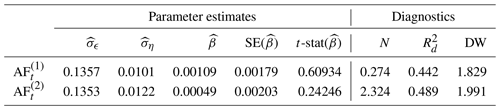We report parameter estimates for the standard deviations σϵ and ση and slope coefficient β together with its standard error (SE) and t statistic (t-stat). We further report the normality (N) test, the goodness-of-fit statistic ${R}_{D}^{\mathrm{2}}$, and the Durbin–Watson (DW) test statistic for serial correlation; all are computed for the standardized prediction errors ut, which are obtained from the Kalman filter. The normality test N is the χ2 distributed, with 2 degrees of freedom, statistic of , with its 95 % critical value of 5.99; the statistic relies on the sample estimates of skewness and kurtosis of ut. The goodness-of-fit statistic ${R}_{d}^{\mathrm{2}}$ is defined as $\mathrm{1}-ESS/DSS$, where ESS $={\sum }_{t=\mathrm{2}}^{n}{u}_{t}^{\mathrm{2}}$ and DSS $={\sum }_{t=\mathrm{2}}^{n}\left[\left({\mathbit{y}}_{t}-{y}_{t-\mathrm{1}}\right)-m{\right]}^{\mathrm{2}}$ with $m=\left(n-\mathrm{2}{\right)}^{-\mathrm{1}}{\sum }_{t=\mathrm{2}}^{n}\left({\mathbit{y}}_{t}-{y}_{t-\mathrm{1}}\right)$. The DW test statistic is developed by , where also its critical values are tabulated. If DW =2 the sequence ut is serially uncorrelated, if DW <2 there is evidence that the errors ut are positively autocorrelated, and if DW >2 there is evidence that the errors ut are negatively autocorrelated.

The state-space system allows both measures for the airborne fraction, AF${}_{t}^{\left(\mathrm{1}\right)}$ and AF${}_{t}^{\left(\mathrm{2}\right)}$, to be included in a single model with the purpose of improving the quality of the trend estimation and inference. We begin with an “uninformed” system using two different trend components, ${T}_{t}^{\left(\mathrm{1}\right)}$ and ${T}_{t}^{\left(\mathrm{2}\right)}$, both specified as Eq. (6), for the two time series. We have

$\begin{array}{}\text{(11)}& \begin{array}{rl}{\mathbit{y}}_{t}& =\left[\begin{array}{c}{\mathrm{AF}}_{t}^{\left(\mathrm{1}\right)}\\ {\mathrm{AF}}_{t}^{\left(\mathrm{2}\right)}\end{array}\right]=\left[\begin{array}{c}{G}_{t}^{\mathrm{ATM}}/{E}_{t}^{\mathrm{ANT}}\\ \mathrm{1}-\left({S}_{t}^{\mathrm{OCEAN}}+{S}_{t}^{\mathrm{LAND}}\right)/{E}_{t}^{\mathrm{ANT}}\end{array}\right]\\ & =\left[\begin{array}{c}{T}_{t}^{\left(\mathrm{1}\right)}\\ {T}_{t}^{\left(\mathrm{2}\right)}\end{array}\right]+\left[\begin{array}{c}{\mathit{ϵ}}_{t}^{\left(\mathrm{1}\right)}\\ {\mathit{ϵ}}_{t}^{\left(\mathrm{2}\right)}\end{array}\right],\end{array}\end{array}$

where the error terms ${\mathit{ϵ}}_{t}^{\left(i\right)}$, for $i=\mathrm{1},\mathrm{2}$, are correlated and their correlation coefficient can be estimated by the method of maximum likelihood together with the other parameters. The estimation results for this model are presented in panel a of Table 2. The main difference from Table 1 is the inclusion of the estimated correlation matrix for $\left({\mathit{ϵ}}_{t}^{\left(\mathrm{1}\right)},{\mathit{ϵ}}_{t}^{\left(\mathrm{2}\right)}\right)$. The diagnostic test statistics are reasonable. In comparison with the univariate analysis, the goodness-of-fit values for ${R}_{d}^{\mathrm{2}}$ are slightly higher for the multivariate model. Hence we trust the model to be a good representation of the data. Furthermore, the slope is estimated to be positive in both cases (that is $\stackrel{\mathrm{^}}{\mathit{\beta }}>\mathrm{0}$). However, when testing the null hypothesis given in Eq. (10), we cannot reject the hypothesis that the slopes are zero (p values 0.3753 and 0.4895 for the case of ${\mathrm{AF}}_{t}^{\left(\mathrm{1}\right)}$ and ${\mathrm{AF}}_{t}^{\left(\mathrm{2}\right)}$, respectively).

Table 2Multivariate analysis of the airborne fraction.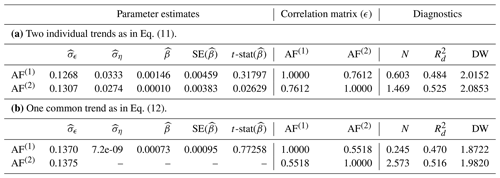We report parameter estimates for the standard deviations ${\mathit{\sigma }}_{\mathit{ϵ}}^{\left(i\right)}$ and ${\mathit{\sigma }}_{\mathit{\eta }}^{\left(i\right)}$, for $i=\mathrm{1},\mathrm{2}$, correlation matrix for ϵt, and slope coefficient β together with its standard error (SE) and t statistic (t-stat). We further report the normality (N) test, the goodness-of-fit statistic ${R}_{D}^{\mathrm{2}}$, and the Durbin–Watson (DW) test statistic for serial correlation. For details, see Table 1. In panel b, the trend coefficients (ση and β) for AF(2) are the same as for AF(1) given the construction of the model (Eq. 12).

Since the two quantities in Eq. (8) measure the same object, the airborne fraction, we now force the state-space system to recognize that these data are driven by the same underlying common trend, ${T}_{t}^{A}$, but with possibly different error terms ${\mathit{ϵ}}_{t}^{\left(\mathrm{1}\right)}$ and ${\mathit{ϵ}}_{t}^{\left(\mathrm{2}\right)}$. In other words, we consider

$\begin{array}{}\text{(12)}& \begin{array}{rl}{\mathbit{y}}_{t}& =\left[\begin{array}{c}{\mathrm{AF}}_{t}^{\left(\mathrm{1}\right)}\\ {\mathrm{AF}}_{t}^{\left(\mathrm{2}\right)}\end{array}\right]=\left[\begin{array}{c}{G}_{t}^{\mathrm{ATM}}/{E}_{t}^{\mathrm{ANT}}\\ \mathrm{1}-\left({S}_{t}^{\mathrm{OCEAN}}+{S}_{t}^{\mathrm{LAND}}\right)/{E}_{t}^{\mathrm{ANT}}\end{array}\right]\\ & =\left[\begin{array}{c}{T}_{t}^{A}\\ {T}_{t}^{A}\end{array}\right]+\left[\begin{array}{c}{\mathit{ϵ}}_{t}^{\left(\mathrm{1}\right)}\\ {\mathit{ϵ}}_{t}^{\left(\mathrm{2}\right)}\end{array}\right].\end{array}\end{array}$

The output of the estimation of this system is shown in panel b of Table 2; the estimated common trend and the data are plotted in Fig. 1. A slight deterioration of the diagnostic statistics is to be expected when introducing a common trend into the system, but the diagnostic statistics are still such that we can accept Eq. (12) as a plausible model. For the estimate of the slope $\stackrel{\mathrm{^}}{\mathit{\beta }}$, we find a larger t statistic in absolute value than in the uninformed model, indicating that the restriction to the common trend increases the precision of the estimates. An explanation of this finding is that the informed system used twice as many observations for estimating the trend compared to the uninformed system. The hypothesis test in Eq. (10) reveals that the estimate of the slope parameter, although again positive, is still not statistically different from zero (p value 0.2199).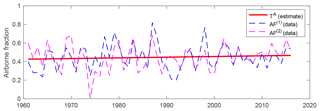Figure 1Estimated trend ${T}_{t}^{A}$ of the airborne fraction from the model 12.

5 Trend analysis of the CO2 sink rate

In this section, we analyse the CO2 sink rate in the same way as the airborne fraction above. Analogously we can define two alternative versions of the sink rate:

$\begin{array}{}\text{(13)}& {k}_{S,t}^{\left(\mathrm{1}\right)}=\frac{{S}_{t}^{O}+{S}_{t}^{L}}{{C}_{t}},\phantom{\rule{2em}{0ex}}{k}_{S,t}^{\left(\mathrm{2}\right)}=\frac{{E}_{t}^{\mathrm{ANT}}-{G}_{t}}{{C}_{t}}={k}_{\mathrm{S},t}^{\left(\mathrm{1}\right)}+{\mathit{\xi }}_{t},\end{array}$

where now ${\mathit{\xi }}_{t}={B}_{t}^{\mathrm{IM}}/{C}_{t}$ and where we used Eq. (2). As was the case for the airborne fraction, these two quantities measure the same underlying object (the sink rate, kS,t) but differ in practice because of a non-zero budget imbalance, i.e. ξt≠0.

The basic (univariate) local linear trend model for each of these objects is then given by

${y}_{t}={k}_{S,t}^{\left(i\right)}={T}_{t}^{\left(i\right)}+{\mathit{ϵ}}_{t}^{\left(i\right)},$

for $i=\mathrm{1},\mathrm{2}$, where ${T}_{t}^{\left(i\right)}$ is specified as in Eq. (6). When the model is cast in the state-space system, the parameters can be estimated for each of the data series individually. The estimation results are presented in Table 3. The diagnostic statistics are satisfactory, and we conclude again that the error term ${\mathit{\xi }}_{t}={B}_{t}^{\mathrm{IM}}/{C}_{t}$ is well-behaved in the sense that the assumptions underlying the state-space system appear to be valid also for the alternative sink rate data, ${k}_{\mathrm{S},t}^{\left(\mathrm{2}\right)}$. Even though the estimates of the slopes are negative, we cannot reject the null hypothesis of β=0 (p values 0.2233 and 0.0761 for the case of ${k}_{S,t}^{\left(\mathrm{1}\right)}$ and ${k}_{S,t}^{\left(\mathrm{2}\right)}$, respectively). We still consider a one-sided test as in Eq. (10), but now the relevant alternative hypothesis is ${H}_{\mathrm{1}}:\mathit{\beta }<\mathrm{0}$.

Table 3Univariate analysis of the CO2 sink rate.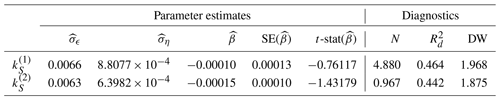We report parameter estimates for the standard deviations σϵ and ση and slope coefficient β together with its standard error (SE) and t statistic (t-stat). We further report the normality (N) test, the goodness-of-fit statistic ${R}_{D}^{\mathrm{2}}$, and the Durbin–Watson (DW) test statistic for serial correlation; all are computed for the standardized prediction errors ut, which are obtained from the Kalman filter; for details see Table 1.

Analogously to the airborne fraction above, these data can be put in a joint uninformed system with two different trend components, and we have

$\begin{array}{}\text{(14)}& {\mathbit{y}}_{t}=\left[\begin{array}{c}{k}_{S,t}^{\left(\mathrm{1}\right)}\\ {k}_{S,t}^{\left(\mathrm{2}\right)}\end{array}\right]=\left[\begin{array}{c}\left({S}_{t}^{O}+{S}_{t}^{L}\right)/{C}_{t}\\ \left({E}_{t}^{\mathrm{ANT}}-{G}_{t}\right)/{C}_{t}\end{array}\right]=\left[\begin{array}{c}{T}_{t}^{\left(\mathrm{1}\right)}\\ {T}_{t}^{\left(\mathrm{2}\right)}\end{array}\right]+\left[\begin{array}{c}{\mathit{ϵ}}_{t}^{\left(\mathrm{1}\right)}\\ {\mathit{ϵ}}_{t}^{\left(\mathrm{2}\right)}\end{array}\right],\end{array}$

which can be compared with the model in Eq. (11). The estimation results for this model are reported in panel a of Table 4. Although the slope estimates are negative, they are not significant (p values 0.3106 and 0.1947 for the case of ${k}_{S,t}^{\left(\mathrm{1}\right)}$ and ${k}_{S,t}^{\left(\mathrm{2}\right)}$, respectively).

Table 4Multivariate analysis of the CO2 sink rate.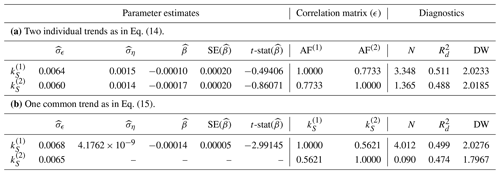We report parameter estimates for the standard deviations ${\mathit{\sigma }}_{\mathit{ϵ}}^{\left(i\right)}$ and ${\mathit{\sigma }}_{\mathit{\eta }}^{\left(i\right)}$, for $i=\mathrm{1},\mathrm{2}$, the correlation matrix for ϵt, and slope coefficient β together with its standard error (SE) and t statistic (t-stat). We further report the normality (N) test, the goodness-of-fit statistic ${R}_{D}^{\mathrm{2}}$, and the Durbin–Watson (DW) test statistic for serial correlation; for details, see Table 1. In panel b, the trend coefficients (ση and β) for ${k}_{S}^{\left(\mathrm{2}\right)}$ are the same as for ${k}_{S}^{\left(\mathrm{1}\right)}$ given the construction of the model (Eq. 15).

Finally, we consider the state-space system that imposes a common trend for both time series, ${T}_{t}^{S}$, that is,

$\begin{array}{}\text{(15)}& {\mathbit{y}}_{t}=\left[\begin{array}{c}{k}_{S,t}^{\left(\mathrm{1}\right)}\\ {k}_{S,t}^{\left(\mathrm{2}\right)}\end{array}\right]=\left[\begin{array}{c}\left({S}_{t}^{O}+{S}_{t}^{L}\right)/{C}_{t}\\ \left({E}_{t}^{\mathrm{ANT}}-{G}_{t}\right)/{C}_{t}\end{array}\right]=\left[\begin{array}{c}{T}_{t}^{S}\\ {T}_{t}^{S}\end{array}\right]+\left[\begin{array}{c}{\mathit{ϵ}}_{t}^{\left(\mathrm{1}\right)}\\ {\mathit{ϵ}}_{t}^{\left(\mathrm{2}\right)}\end{array}\right],\end{array}$

which can be compared with model in Eq. (12). The estimation results are presented in panel b of Table 4. Similar to the analysis of the airborne fraction in the previous section, the diagnostic statistics are somewhat worse for the less flexible system with a common trend. However, the diagnostics are still satisfactory, while the goodness-of-fit statistics improved overall. The estimate of the slope is

$\stackrel{\mathrm{^}}{\mathit{\beta }}=-\mathrm{0.00014},$

and this estimate is statistically significant: we reject the hypothesis ${H}_{\mathrm{0}}:\mathit{\beta }=\mathrm{0}$ in favour of ${H}_{\mathrm{1}}:\mathit{\beta }<\mathrm{0}$ at a 95 % confidence level (p value 0.0014). The mean of the sink rate (calculated using either data set ${k}_{S}^{\left(\mathrm{1}\right)}$ or ${k}_{S}^{\left(\mathrm{2}\right)}$) is 0.0258. It follows that we estimate the sink rate to be decreasing with approximately $\mathrm{0.00014}/\mathrm{0.0258}=\mathrm{0.54}\phantom{\rule{0.125em}{0ex}}\mathit{%}$ yr−1. The estimated trend and the data are plotted in Fig. 2.

The state-space system is also well-suited for forecasting; see . Using the model in Eq. (15), we forecast the sink rate 25 years ahead in time. The output is presented in Fig. 3. For reference, the forecasts coming from an autoregressive model of order 1 (AR1) are also presented. The downward trend in the forecasts from the state-space model is the result of the negative estimate of β. Under current conditions, the forecast implies that in approximately 15 years, the sink rate will have declined to below 2 %. Conversely, the autoregressive model produces forecasts that converge to the mean of the original data series.

It is important to recognize that the validity of these forecasts are conditional on the assumption that the sink rate, kS, is linear in concentrations. As seen from the analysis above, see also Fig. 2, this assumption has been approximately satisfied over the time period considered in this paper, but whether it will continue to be accurate is an open question (see Appendix A for a discussion of the possible future behaviour of the sink rate). The model-based forecasts of Fig. 3 should be seen in this light: these forecasts are obtained under the assumption that the sink rate will continue to be approximately linear in concentrations. Whether this assumption is reasonable is an interesting question beyond the scope of the present study.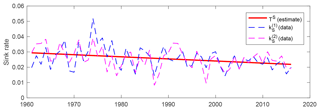Figure 2Estimated trend ${T}_{t}^{S}$ of the CO2 sink rate from Model (Eq. 15).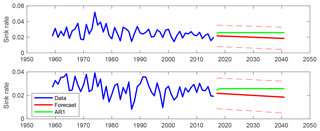Figure 3The blue solid line represents the data, while the red solid line represents the point forecasts from the Kalman filter with the unknown parameters estimated by maximum likelihood. The dashed red lines are 95 % confidence bands (±1.96 standard deviation) for the forecasts. The green line represents the forecasts from an autoregressive model of order 1.

6 Trend analysis of the ocean and land sink rates

We may conclude from the analysis in the previous section that the combined (land plus ocean) sink rate appears to be decreasing. To investigate this finding in more detail, we study two alternative models, which consider the two sink variables separately. The first model specifies local linear trends for ocean and land sink rates, i.e.

$\begin{array}{}\text{(16)}& {\mathbit{y}}_{t}=\left[\begin{array}{c}{k}_{O,t}\\ {k}_{L,t}\end{array}\right]=\left[\begin{array}{c}{S}_{t}^{O}/{C}_{t}\\ {S}_{t}^{L}/{C}_{t}\end{array}\right]=\left[\begin{array}{c}{T}_{t}^{O}\\ {T}_{t}^{L}\end{array}\right]+\left[\begin{array}{c}{\mathit{ϵ}}_{t}^{\left(\mathrm{1}\right)}\\ {\mathit{ϵ}}_{t}^{\left(\mathrm{2}\right)}\end{array}\right],\end{array}$

where the time series kO,t and kL,t are defined in Eq. (4), while the trend variables ${T}_{t}^{O}$ and ${T}_{t}^{L}$ are specified as in Eq. (6). To inform the state-space system of the structure of the carbon budget, we also consider the model equations

$\begin{array}{}\text{(17)}& \begin{array}{rl}{\mathbit{y}}_{t}& =\left[\begin{array}{c}{k}_{O,t}\\ {k}_{L,t}\\ {k}_{\mathrm{S},t}\end{array}\right]=\left[\begin{array}{c}{S}_{t}^{O}/{C}_{t}\\ {S}_{t}^{L}/{C}_{t}\\ \left({E}_{t}^{\mathrm{ANT}}-{G}_{t}\right)/{C}_{t}\end{array}\right]=\left[\begin{array}{c}{T}_{t}^{O}\\ {T}_{t}^{L}\\ {T}_{t}^{O}+{T}_{t}^{L}\end{array}\right]\\ & +\left[\begin{array}{c}{\mathit{ϵ}}_{t}^{\left(\mathrm{1}\right)}\\ {\mathit{ϵ}}_{t}^{\left(\mathrm{2}\right)}\\ {\mathit{ϵ}}_{t}^{\left(\mathrm{3}\right)}\end{array}\right].\end{array}\end{array}$

This trivariate model equation can be cast in the state-space system (Eq. 5) as well. The model specification has two independent trend processes of the form Eq. (6) for land and ocean sinks. Since ${k}_{\mathrm{S},t}={k}_{O,t}+{k}_{L,t}$, the time series kS,t of combined ocean and land sinks must feature the sum of the two trend processes for the individual sinks as its trend process.

The estimation results for these two model specifications are presented in Table 5. The residual diagnostic statistics N and DW are satisfactory, but we are particularly interested in the estimates of the slope parameters. It seems that most of the decrease in the sink rate can be attributed to the land sink. The slope estimates of the trend driving the ocean sink rate are very close to zero and not statistically significant (p values 0.5227 and 0.5168, respectively). On the other hand, the slope estimates of the trend driving the land sink rate are negative for both specifications. In the first model (Eq. 16), we can reject the hypothesis that the slope of the trend driving the land sink rate is zero in favour of the one-sided alternative ${H}_{\mathrm{1}}:\mathit{\beta }<\mathrm{0}$ at a 95 % confidence level (p value of 0.0420). For the more informed model specification (Eq. 17), the estimation results are reported in panel b of Table 5. Here we can reject H0 at a 90 % confidence level (p value of 0.0882). Further, the results show that the estimate of the slope parameter from the land sink rate is equal to the estimate of the slope parameter from the combined sink rate as in Sect. 5, that is, $\stackrel{\mathrm{^}}{\mathit{\beta }}=-\mathrm{0.00014}$. In other words, it appears that the decrease in the combined sink rate studied in the previous section is entirely explained by the decrease in the land sink rate.

Table 5Analysis of ocean and land sink rates.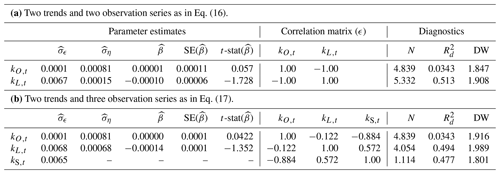We report parameter estimates for standard deviations ${\mathit{\sigma }}_{\mathit{ϵ}}^{\left(i\right)}$ and ${\mathit{\sigma }}_{\mathit{\eta }}^{\left(i\right)}$, for $i=\mathrm{1},\mathrm{2},\mathrm{3}$, correlation matrix for ϵt, and slope coefficient β together with its standard error (SE) and t statistic (t-stat). We further report the normality (N) test, the goodness-of-fit statistic ${R}_{D}^{\mathrm{2}}$ and the Durbin–Watson (DW) test statistic for serial correlation; for details see Table 1. In panel b, we have two trends and two sets of trend coefficients (ση and β) for kO,t and kL,t. The trend for kS,t is a combination of the two given the construction of the model (Eq. 17).

7 Discussion

Previous studies of the airborne fraction and the CO2 sink rate have focused on detecting a single linear and deterministic trend in the data of the form a0+a1t, where a0 and a1, are constants . However, possible statistical difficulties in such analyses have been pointed out in . For instance, a linear regression analysis of two or more non-stationary variables can yield invalid inference . The approach of this paper is to consider the data in a state-space system. In this way, non-stationary components are explicitly modelled as unobserved trend components and inference is valid (e.g. Durbin and Koopman2012). Furthermore, the trend specification of the state-space system allows for both deterministic and stochastic trend components.

In some of the uninformed models (cf. Table 1, panel a of Table 2, and panel a of Table 4), we estimate ${\stackrel{\mathrm{^}}{\mathit{\sigma }}}_{Slp}>\mathrm{0}$, and, thus, in these cases, we find evidence of the trend component varying in time. However, in our “informed” models with a single trend object for two alternative time series, the extracted trends are practically deterministic, that is, the estimates of σSlp in panel b of Tables 2 and 4 are near zero (cf. also Figs. 1 and 2). In conclusion, there is evidence that a simple deterministic trend fits both the airborne fraction and the sink rate data well, although this only becomes evident when incorporating two data sets for each of these objects.

Several studies have highlighted the need for accounting for noise in measurements of climate-related data . The state-space approach explicitly incorporates such noise in the framework as well. argue that errors in ${E}_{t}^{\mathrm{ANT}}$ might be autocorrelated. As shown in Tables 15, the diagnostic statistics do not indicate that autocorrelated errors pose a serious problem in our specifications. Nevertheless, the state-space framework can incorporate autocorrelated errors in the measurement equation.

Why do we find statistical evidence of a decreasing CO2 sink rate but no evidence of an increasing airborne fraction when these two quantities are closely linked and the data entering the analyses are the same? It was noted in that the airborne fraction and the sink rate are actually not as closely linked as they may seem prima facie. In particular, an increasing airborne fraction does not necessarily imply a decreasing sink rate and vice versa (Gloor et al.2010, Section 8). The findings of this paper support this claim by providing statistical evidence for a constant airborne fraction but at the same time for a decreasing sink rate. Secondly, the concept of an airborne fraction is that of a long-term quantity: the airborne fraction should represent the amount of anthropogenically released CO2 that eventually stays in the atmosphere after other fluxes have been taken into account. However, the ratio of the concurrent fluxes, i.e. ${G}_{t}/{E}_{t}^{\mathrm{ANT}}$, is likely a very noisy measurement of this object. Also, as we saw above, it is reasonable to consider sink fluxes, and therefore indirectly Gt, as being dependent on the level of CO2 in the atmosphere (i.e. ${C}_{t}=\sum {G}_{t}$), which is not captured by the concurrent ratio ${G}_{t}/{E}_{t}^{\mathrm{ANT}}$. When studying the airborne fraction, it would perhaps be more reasonable to study an object taking this cumulative nature into account, e.g. $\sum {G}_{t}/\sum {E}_{t}^{\mathrm{ANT}}={C}_{t}/\sum {E}_{t}^{\mathrm{ANT}}$ (in fact, such specifications were often considered in earlier parts of the literature; e.g. Keeling1973; Bacastow and Keeling1979; Oeschger and Heimann1983; Enting and Pearman1986). However, cumulative statistics of this type would present other difficulties. The dominance of the long-term history may mask sudden changes, for example. In contrast, the sink rate StCt, as a flow-to-stock ratio, is immediately compatible with the underlying theory, at least as long as the linear approximation of the relationship between St and Ct, such as was made in, for example, and , is adequate.

What are possible physical explanations for the apparent decrease in the sink rate? argues that a necessary condition for a constant sink rate is that the so-called “LinExp” assumption holds, i.e. that the sink fluxes ${S}_{t}^{O}$ and ${S}_{t}^{L}$ are linear in concentrations Ct (“Lin”) and that emissions (${E}_{t}^{\mathrm{ANT}}$) grow exponentially (“Exp”). Constancy of the airborne fraction rests on a similar LinExp argument. Since we find no statistical evidence that the airborne fraction, AFt, and the ocean sink rate, kO,t, are non-constant in time, it is unlikely that the Exp assumption is grossly violated over the observation period considered in this paper. In contrast, it was found above that the efficiency of the land sink, kL,t, is decreasing. A plausible explanation of these findings is that the Lin assumption no longer holds for the land sink, for instance because the terrestrial sink could be slowly saturating . In Appendix A we give a formal argument for how this could lead to the findings documented above. In particular, we show that the findings of this paper can be explained by the land sink's response to high atmospheric CO2 concentrations: it is plausible that due to a rising level of CO2 concentration, non-linear effects in the terrestrial CO2 carbon cycle have become noticeable. If this is indeed the case, it has obvious consequences for our understanding of the carbon cycle and should be a cause for substantial concern (Gloor et al.2010, p. 7740). However, although this explanation of our findings is consistent with the data, we can not conclude that it is the only possible explanation. Further research into the underlying reasons for the decreasing sink rate would be very valuable and is left for future work.

It is possible that the analyses conducted above are influenced by external natural events such as the El Niño–Southern Oscillation (ENSO), volcanic eruptions, and the like . The state-space system used in this paper can explicitly account for such effects through the additive error terms ϵt (cf. Eq. 5). To verify that the approach is indeed robust to such external and transitory events, we have also conducted our analyses using 5-year average data. The findings from the estimated state-space system for these time series of averages confirm those reported above: in the joint estimation, we find no statistical evidence of a trend in the airborne fraction (p value of 0.3214), and we do find statistical evidence of a decreasing trend in the sink rate (p value of 0.00064). We conclude that the findings of this paper are not likely to be driven by external natural events such as ENSO and volcanic eruptions. We also considered 2-, 3-, and 4-year averages with similar results. We present details of this analysis in the Supplement (Sect. 3). To further check the robustness of the results, we examined whether there are any observations in the data set which are particularly influential. Statistically influential observations could be due to outliers, caused for instance by external natural events, such as the ones mentioned above. Using a statistic called Cook's distance , which is a measure of how influential a given observation is on the analysis, we did not find evidence of any one observation being particularly influential. Similarly, we tried estimating the slope parameter β after deleting the tth observation for each time point t in the sample, i.e. for $t=\mathrm{1959},\mathrm{1960},\mathrm{\dots },\mathrm{2016}$; the estimates of the slope parameter found in this way were very stable, which is further evidence of the robustness of the analyses to potential outliers and external events. Details can be found in the Supplement (Sect. 2.1).

This paper considers data recorded at a yearly frequency, while many of the previous studies of the airborne fraction and the sink rate use monthly data. The advantage of using monthly data is obvious: more observations. However, there are also some disadvantages. For instance, while the CO2 concentration Ct (and therefore also the growth rate Gt) is recorded every month, these data contain a strong seasonal component induced by the photosynthesis–respiration cycle of terrestrial vegetation. This seasonality needs to be accounted for in some way; for instance, smooth the data using a 15-month running mean. In contrast, some of the other data are recorded only yearly, for instance, the emission data available to us, ${E}_{t}^{\mathrm{ANT}}$. In this case, use linear interpolation to get monthly estimates of emissions. Such transformations of the data, i.e. smoothing or interpolation, might introduce new and complicated errors, possibly invalidating the analyses. For these reasons, we prefer to work with yearly data.

8 Conclusions

We have argued that the state-space system can be a useful approach for analysing possible trends in the airborne fraction of anthropogenically released CO2 and in the CO2 sink rate. We have shown that deterministic and stochastic trend processes can be explicitly and jointly incorporated as unobserved components, allowing for valid inference, even when the observed time series are non-stationary. The state-space framework also allows for the incorporation of multiple data sets for the same object, which increases reliability of the resulting estimates.

We estimate a positive, yet statistically insignificant, slope in the data for the airborne fraction. Using two alternative time series for the sink rate and imposing a common trend, we obtain a significantly negative deterministic trend. Our analyses support the conclusions as set out by : the rate at which the combined (ocean plus land) sink takes up CO2 from the atmosphere seems to be decreasing. The best estimate resulting from our state-space system is that the CO2 sink rate, and therefore the efficiency with which the combined land and ocean sink is absorbing carbon from the atmosphere, is decreasing by 0.54 % yr−1. We do not find evidence of this rate itself changing over time.

Finally, there is tentative evidence that the decrease in the sink rate is mainly driven by a weakening uptake in the land sink. This could be the result of non-linearities in the response of the terrestrial carbon sink to the level of atmospheric concentrations of CO2. That is, although the land sink is itself increasing and thus continuing to take up a large part of anthropogenically emitted CO2, as also noted recently by, for example, , , and , the rate of this uptake appears to be decreasing. The statistical evidence for this is not strong, however, and we suggest that additional research must be conducted to further investigate this question.

Data availability

The data used in this paper are available at the website of the Global Carbon Project (https://www.globalcarbonproject.org, Le Quéré et al., 2018).

Appendix A: Linear approximation of the relation of land sink and concentrations

In this Appendix, we argue that the levels of atmospheric concentrations of CO2 may have risen to a point where a linear expansion of the logarithmic formula, describing the flux of CO2 into the land sink, is no longer sufficient. Consequently, the Lin assumption of might be violated for the land sink, implying that 2nd-order effects may be driving the negative slope of the sink rate that we document in this paper.

From Eq. (4) we obtain the relation

${S}_{t}^{L}={k}_{L,t}\cdot {C}_{t},$

which implies that the flux of CO2 to the land sink is linear in Ct, where kL,t would then be treated as a constant. On the other hand, a decreasing kL,t implies that the efficiency with which the land sink absorbs CO2 is decreasing, i.e. that the flux of CO2 to the land sink is non-linear in Ct and that this non-linearity is such that the efficiency is decreasing. These statements are consistent with simulation results from climate cycle models . Here we illustrate mathematically how such non-linearities can arise.

The precise relationship between ${S}_{t}^{L}$ and Ct still eludes us, but (, p. 94) suggest that (in our notation)

${S}_{t}^{L}\approx \mathit{\alpha }\mathrm{log}\left(\mathrm{1}+{C}_{t}/{\mathcal{C}}^{\mathrm{0}}\right),$

where α is a constant and 𝒞0=591.30 GtC is the amount of CO2 in the atmosphere in pre-industrial times. Using this function, we can write a 2nd-order Taylor expansion:

${S}_{t}^{L}\approx \mathit{\alpha }\mathrm{log}\left(\mathrm{1}+{C}_{t}/{\mathcal{C}}^{\mathrm{0}}\right)\approx \mathit{\alpha }\frac{{C}_{t}}{{\mathcal{C}}^{\mathrm{0}}}-\frac{\mathrm{1}}{\mathrm{2}}\mathit{\alpha }{\left(\frac{{C}_{t}}{{\mathcal{C}}^{\mathrm{0}}}\right)}^{\mathrm{2}}.$

Thus, if 𝒞0 is large compared to Ct, this implies that the squared term in the above equation is small and thus that a linear specification between ${S}_{t}^{L}$ and Ct is reasonable. However, once Ct becomes large compared to 𝒞0, this shows that the estimated sink rate will be found to be decreasing. To see this, use the Taylor expansion to write

${S}_{t}^{L}\approx {k}_{L,t}{C}_{t},$

where

${k}_{L,t}=\frac{\mathit{\alpha }}{{\mathcal{C}}^{\mathrm{0}}}-\frac{\mathrm{1}}{\mathrm{2}}\frac{\mathit{\alpha }}{{\mathcal{C}}^{\mathrm{0}}}\frac{{C}_{t}}{{\mathcal{C}}^{\mathrm{0}}},$

is decreasing in Ct. In our data, we have C1959≈80 GtC and C2016≈267 GtC, resulting in ${C}_{\mathrm{1959}}/{\mathcal{C}}^{\mathrm{0}}\approx \mathrm{14}\phantom{\rule{0.125em}{0ex}}\mathit{%}$ and ${C}_{\mathrm{2016}}/{\mathcal{C}}^{\mathrm{0}}\approx \mathrm{45}\phantom{\rule{0.125em}{0ex}}\mathit{%}$. In other words, the linear approximation to the Bacastow and Keeling model of the land sink flux might have been reasonable in the past, since ${C}_{\mathrm{1959}}/{\mathcal{C}}^{\mathrm{0}}\approx \mathrm{14}\phantom{\rule{0.125em}{0ex}}\mathit{%}$, but is likely misspecified in the present, since ${C}_{\mathrm{2016}}/{\mathcal{C}}^{\mathrm{0}}\approx \mathrm{45}\phantom{\rule{0.125em}{0ex}}\mathit{%}$. That is, if this model is accurate, then a decreasing (land) sink rate indicates that we have entered a regime of atmospheric CO2 concentrations, where the linear approximation breaks down and higher-order terms should be taken into account.

Supplement

Author contributions

MB, EH, and SJK studied the data and discussed possible models. MB conceived of the idea to focus on the airborne fraction and the sink rate. MB, EH, and SJK went through the modelling cycle for these objects of interest. MB and SJK ran the estimations in MATLAB and OX, respectively. MB, EH, and SJK discussed the results and wrote the paper jointly.

Competing interests

The author declares that there is no conflict of interest.

Acknowledgements

We would like to thank Corinne Le Quéré for permission to use the data set of as well as for useful comments on the paper. We would also like to thank two anonymous referees and the associate editor for constructive and helpful comments.

Financial support

This research has been supported by the Independent Research Fund Denmark (grant no. 7015-00018B).

Review statement

This paper was edited by Laurent Bopp and reviewed by two anonymous referees.

References

Atkinson, A. C., Koopman, S. J., and Shephard, N.: Detecting shocks: Outliers and breaks in time series, J. Econometrics, 80, 387–422, 1997. a

Bacastow, R. and Keeling, C. D.: Atmospheric Carbon Dioxide and radiocarbon in the natural cycle: II. Changes from A. D. 1700 to 2070 as deduced from a geochemical model, in: Carbon and the biosphere conference proceedings; Upton, New York, USA, 86–135, Brookhaven Symposia in Biology, 1973. a, b

Bacastow, R. B. and Keeling, C. D.: Models to predict future atmospheric CO2 concentrations, in: Workshop on the global effects of carbon dioxide from fossil fuels, 72–90, US Department of Energy, 1979. a

Ballantyne, A. P., Andres, R., Houghton, R., Stocker, B. D., Wanninkhof, R., Anderegg, W., Cooper, L. A., DeGrandpre, M., Tans, P. P., Miller, J. B., Alden, C., and White, J. W. C.: Audit of the global carbon budget: estimate errors and their impact on uptake uncertainty, Biogeosciences, 12, 2565–2584, https://doi.org/10.5194/bg-12-2565-2015, 2015. a, b, c, d, e

Boden, T. A., Marland, G., and Andres, R. J.: Global, Regional, and National Fossil-Fuel CO2 Emissions, oak Ridge National Laboratory, U.S. Department of Energy, Oak Ridge, Tenn., USA, available at: http://cdiac.ornl.gov/trends/emis/overview_2014.html (last access: 28 June 2017), 2018. a

Canadell, J. G., Le Quéré, C., Raupach, M. R., Field, C. B., Buitenhuis, E. T., Ciais, P., Conway, T. J., Gillett, N. P., Houghton, R. A., and Marland, G.: Contributions to accelerating atmospheric CO2 growth from economic activity, carbon intensity, and efficiency of natural sinks, P. Natl. Acad. Sci. USA, 104, 18866–18870, https://doi.org/10.1073/pnas.0702737104, 2007a. a, b

Canadell, J. G., Pataki, D., Gifford, R., Houghton, R., Luo, Y., Raupach, M., Smith, P., and Steffen, W.: Saturation of the Terrestrial Carbon Sink, 59–78, https://doi.org/10.1007/978-3-540-32730-1_6, 2007b. a

Cook, R. D.: Detection of influential observations in linear regression, Technometrics, 19, 15–18, 1977. a

Cook, R. D.: Influential observations in linear regression, J. Am. Stat. Assoc., 74, 169–174, 1979. a

Dlugokencky, E. and Tans, P.: Trends in atmospheric carbon dioxide, national Oceanic & Atmospheric Administration, Earth System Research Laboratory (NOAA/ESRL), available at: http://www.esrl.noaa.gov/gmd/ccgg/trends/global.html last access: 9 March 2018. a

Durbin, J. and Koopman, S. J.: Time series analysis by state space methods, 38, Oxford University Press, 2012. a, b, c, d, e

Durbin, J. and Watson, G. S.: Testing for Serial Correlation in Least Squares Regression, Biometrika, 58, 1–19, 1971. a, b

Enting, I. G. and Pearman, G. I.: The Use of Observations in Calibrating and Validating Carbon Cycle Models, 425–458, Springer New York, New York, NY, https://doi.org/10.1007/978-1-4757-1915-4_21, 1986. a

Fernández-Martínez, M., Sardans, J., Chevallier, F., Ciais, P., Obersteiner, M., Vicca, S., Canadell, J. G., Bastos, A., Friedlingstein, P., Sitch, S., Piao, S. L., Janssens, I. A., and Peñuelas, J.: Global trends in carbon sinks and their relationships with CO2 and temperature, Nat. Clim. Change, 9, 73–79, https://doi.org/10.1038/s41558-018-0367-7, 2019. a

Friedlingstein, P., Cox, P., Betts, R., Bopp, L., von Bloh, W., Brovkin, V., Cadule, P., Doney, S., Eby, M., Fung, I., Bala, G., John, J., Jones, C., Joos, F., Kato, T., Kawamiya, M., Knorr, W., Lindsay, K., Matthews, H. D., Raddatz, T., Rayner, P., Reick, C., Roeckner, E., Schnitzler, K.-G., Schnur, R., Strassmann, K., Weaver, A. J., Yoshikawa, C., and Zeng, N.: Climate–Carbon Cycle Feedback Analysis: Results from the C4MIP Model Intercomparison, J. Climate, 19, 3337–3353, https://doi.org/10.1175/JCLI3800.1, 2006. a

Frölicher, T. L., Joos, F., Raible, C. C., and Sarmiento, J. L.: Atmospheric CO2 response to volcanic eruptions: The role of ENSO, season, and variability, Global Biogeochem. Cy., 27, 239–251, https://doi.org/10.1002/gbc.20028, 2013. a

Gloor, M., Sarmiento, J. L., and Gruber, N.: What can be learned about carbon cycle climate feedbacks from the CO2 airborne fraction?, Atmos. Chem. Phys., 10, 7739–7751, https://doi.org/10.5194/acp-10-7739-2010, 2010. a, b, c, d, e, f, g

Granger, C. W. J. and Newbold, P.: Spurious regression in econometrics, J. Econometrics, 2, 111–120, 1974. a

Hansis, E., Davis, S. J., and Pongratz, J.: Relevance of methodological choices for accounting of land use change carbon fluxes, Global Biogeochem. Cy., 29, 1230–1246, 2015. a

Houghton, R. A. and Nassikas, A. A.: Global and regional fluxes of carbon from land use and land cover change 1850-2015, Global Biogeochem. Cy., 31, 456–472, 2017. a

Jarque, C. M. and Bera, A. K.: A Test for Normality of Observations and Regression Residuals, Int. Stat. Rev., 2, 163–172, 1987. a, b

Keeling, C. D.: The Carbon Dioxide Cycle: Reservoir Models to Depict the Exchange of Atmospheric Carbon Dioxide with the Oceans and Land Plants, 251–329, Springer US, Boston, MA, https://doi.org/10.1007/978-1-4684-1986-3_6, 1973. a

Keenan, T. F., Prentice, I. C., Canadell, J. G., Williams, C. A., Wang, H., Raupach, M., and Collatz, G. J.: Recent pause in the growth rate of atmospheric CO2 due to enhanced terrestrial carbon uptake, Nat. Commun., 7, 13428, https://doi.org/10.1038/ncomms13428, 2016. a

Knorr, W.: Is the airborne fraction of anthropogenic CO2 emissions increasing?, Geophys. Res. Lett., 36, L21710, https://doi.org/10.1029/2009GL040613, 2009. a, b, c, d, e, f

Le Quéré, C., Raupach, M. R., Canadell, J. G., Marland, G., Bopp, L., Ciais, P., Conway, T. J., Doney, S. C., Feely, R. A., Foster, P., Friedlingstein, P., Gurney, K., Houghton, R. A., House, J. I., Huntingford, C., Levy, P. E., Lomas, M. R., Majkut, J., Metzl, N., Ometto, J. P., Peters, G. P., Prentice, I. C., Randerson, J. T., Running, S. W., Sarmiento, J. L., Schuster, U., Sitch, S., Takahashi, T., Viovy, N., van der Werf, G. R., and Woodward, F. I.: Trends in the sources and sinks of carbon dioxide, Nat. Geosci., 2, 831–836, 2009. a, b

Le Quéré, C., Andrew, R. M., Friedlingstein, P., Sitch, S., Pongratz, J., Manning, A. C., Korsbakken, J. I., Peters, G. P., Canadell, J. G., Jackson, R. B., Boden, T. A., Tans, P. P., Andrews, O. D., Arora, V. K., Bakker, D. C. E., Barbero, L., Becker, M., Betts, R. A., Bopp, L., Chevallier, F., Chini, L. P., Ciais, P., Cosca, C. E., Cross, J., Currie, K., Gasser, T., Harris, I., Hauck, J., Haverd, V., Houghton, R. A., Hunt, C. W., Hurtt, G., Ilyina, T., Jain, A. K., Kato, E., Kautz, M., Keeling, R. F., Klein Goldewijk, K., Körtzinger, A., Landschützer, P., Lefèvre, N., Lenton, A., Lienert, S., Lima, I., Lombardozzi, D., Metzl, N., Millero, F., Monteiro, P. M. S., Munro, D. R., Nabel, J. E. M. S., Nakaoka, S., Nojiri, Y., Padin, X. A., Peregon, A., Pfeil, B., Pierrot, D., Poulter, B., Rehder, G., Reimer, J., Rödenbeck, C., Schwinger, J., Séférian, R., Skjelvan, I., Stocker, B. D., Tian, H., Tilbrook, B., Tubiello, F. N., van der Laan-Luijkx, I. T., van der Werf, G. R., van Heuven, S., Viovy, N., Vuichard, N., Walker, A. P., Watson, A. J., Wiltshire, A. J., Zaehle, S., and Zhu, D.: Global Carbon Budget 2017, Earth Syst. Sci. Data, 10, 405–448, https://doi.org/10.5194/essd-10-405-2018, 2018.  a, b, c, d, e, f

Oeschger, H. and Heimann, M.: Uncertainties of predictions of future atmospheric CO2 concentrations, J. Geophys. Res.-Oceans, 88, 1258–1262, https://doi.org/10.1029/JC088iC02p01258, 1983. a

Raupach, M. R.: The exponential eigenmodes of the carbon-climate system, and their implications for ratios of responses to forcings, Earth Syst. Dynam., 4, 31–49, https://doi.org/10.5194/esd-4-31-2013, 2013. a, b, c, d

Raupach, M. R., Canadell, J. G., and Le Quéré, C.: Anthropogenic and biophysical contributions to increasing atmospheric CO2 growth rate and airborne fraction, Biogeosciences, 5, 1601–1613, https://doi.org/10.5194/bg-5-1601-2008, 2008. a, b

Raupach, M. R., Gloor, M., Sarmiento, J. L., Canadell, J. G., Frölicher, T. L., Gasser, T., Houghton, R. A., Le Quéré, C., and Trudinger, C. M.: The declining uptake rate of atmospheric CO2 by land and ocean sinks, Biogeosciences, 11, 3453–3475, https://doi.org/10.5194/bg-11-3453-2014, 2014. a, b, c, d, e, f, g, h, i

Rayner, P. J., Stavert, A., Scholze, M., Ahlström, A., Allison, C. E., and Law, R. M.: Recent changes in the global and regional carbon cycle: analysis of first-order diagnostics, Biogeosciences, 12, 835–844, https://doi.org/10.5194/bg-12-835-2015, 2015. a, b, c

The data are available at http://www.globalcarbonproject.org/ (last access: 1 June 2018).